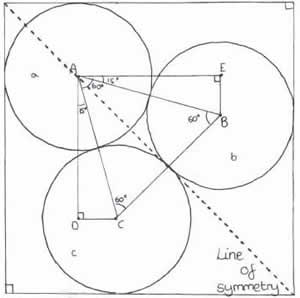#### You may also like### Doodles

Draw a 'doodle' - a closed intersecting curve drawn without taking pencil from paper. What can you prove about the intersections?### Russian Cubes

I want some cubes painted with three blue faces and three red faces. How many different cubes can be painted like that?### Picture Story

Can you see how this picture illustrates the formula for the sum of the first six cube numbers?

# Circle Box

##### Age 14 to 16 Challenge Level:

Many thanks to Hannah of The School of St Helen and St Katherine for submitting this solution. Very well done Hannah.Looking at the diagram of the problem; let the top circle be a, the slightly lower one be b and the bottom one be
c and their centres A, B and C.

Draw a horizontal line to the left of C and extend it to the edge of the square.

Now draw a vertical line down from A and call the point where it meets the line you have just drawn D so that angle ADC = $90^o$

It is also worth noting that triangle ABC is equilateral and the whole box has a line of symmetry running from the top left hand corner of the square to the bottom right.

The angle DAE (on diagram) is $90^o$ and the angle CAB is $60^o$ because it is an equilateral triangle. Therefore angle DAC + angle EAB equals $30^o; (90^o - 60^o)$ meaning that angle DAC is $15^o$; as it equals EAB.

Now we can use trigonometry to work out the length AD (from A, vertically down to D). Using the triangle ADC, the hypotenuse of which is 1cm (two radii), ($90^o \times 1 = 0.965...$)

From C to the base of the square is 0.5cm (radius), which is equal to the distance from D to the base of the square. From A to the top of the square is also 0.5cm (radius)and you can add these two values on to AD to get a side of the square.

Therefore one side of the square $= 0.5+0.5 (a+c$ radii$)+0.965... =1.966$ cm(3d.p.)